### Home > PC3 > Chapter 8 > Lesson 8.1.2 > Problem8-41

8-41.
1. Simplify each of the following rational expressions. Homework Help ✎

1.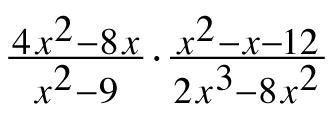2. (x2y2) ÷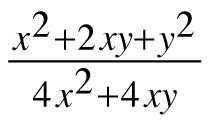3.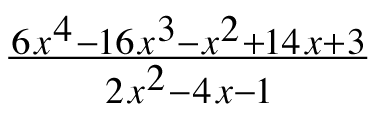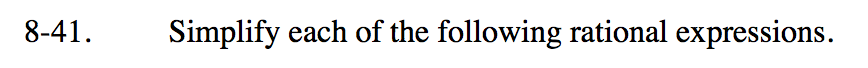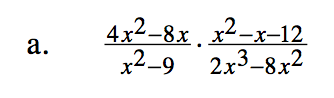Factor all of the expressions. Then simplify.

$\frac{4x(x-2)}{(x-3)(x+3)}\cdot \frac{(x-4)(x+3)}{2x^2(x-4)}$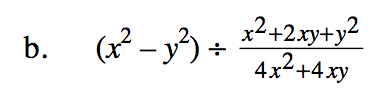Write (x2y2) over 1. Then follow the same process as shown in part (a).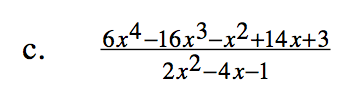Use long division.